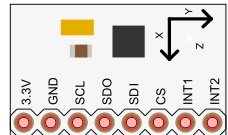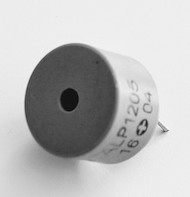# Measuring Acceleration

## Introduction

The accelerometer is a component which measures the change of speed, i.e., acceleration. The component in the Mehackit Maker Kit measures acceleration in three axes, X, Y, and Z.

You can find an accelerometer in many everyday devices, like new smartphones. In practice, by measuring acceleration, you can determine the position of the device!

In this exercise, you’ll learn more about an accelerometer and produce different sounds using accelerations it detects.

## Parts

In addition to Mehackit board (or Arduino), USB cable, breadboard, and wires, you’ll need:

PartPictureDescription
LIS331 AccelerometerThe accelerometer registers acceleration in X, Y, and Z axes, and you can communicate with it using SPI communication.
Piezo-buzzerPiezo is a simple speaker, with which you can turn changes in voltage to sound. Many Piezos can also be used as sensors.

## The Circuit

• As in the picture, connect the accelerometer to 3,3V voltage, to ground, and to pins 10, 11, 12, and 13. Don’t connect the accelerometer to 5V voltage because it may break it!
• Connect the Piezo buzzer to a digital pin and to ground.

## Programming

You’ll find the program to use the accelerometer here. The program is quite long and a bit complicated. However, by reading the comments written in the program, you’ll get a good picture of how it works.

Do This
Upload the program to Arduino. Open the Serial Monitor. First, turn the accelerator around in its place. Try then waving the sensor with accelerating motions in different directions. What are the biggest values you can get from the sensors in x, y, and z directions? Can you tell, what the unit of the values is?

The values are converted to gravitational acceleration (g = 9,81 m/s^2). For example, the value 2 means two gravitational accelerations. When you hold the sensor in its place, the acceleration directing downward should, in practice, be one gravitational acceleration because of gravity. When the sensor is level, the z-axis points downwards.

Which of the following is the best use for en accelerometer?

## Making a Magic Wand

Earlier, you connected the Piezo buzzer. Now, it’s your job to create a program that plays different notes depending on acceleration!

Remove the comment marks ("//") from line 36, where the function magicWand is called. In other words, put the command in line 36 to use! Wave the accelerometer and observe, what kind of sound the Piezo produces.

Edit the magicWand function and see, with what kind of logic can you produce the most interesting sounds. Can you make the Piezo produce a different sound every time the acceleration in x, y, or z direction exceeds the value 2?

Note, that in steady motion the acceleration is 0, so in principle, the Piezo doesn’t make a sound. It’ll only produce sound when the motion is accelerating. However, because gravity produces slight acceleration to the sensor, the Piezo makes a low sound even in “normal state”.

Below is an example of a magic wand! You can make your own or develop the idea further as part of the final project.